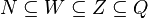# Real number

## Classification

Real numbers are classified as rational numbers (denoted by Q), integers (Z), whole numbers (W), natural numbers, and irrational numbers. In order of inclusion, non-irrational real numbers can be ordered as follows:$N \subseteq W \subseteq Z \subseteq Q$